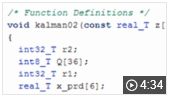# MATLAB Coder 快速入门

MATLAB 代码生成 C 和 C++ 代码

MATLAB® Coder™ 可从 MATLAB 代码生成适用于各种硬件平台（从桌面计算机系统到嵌入式硬件）的 C 和 C++ 代码。它支持大多数 MATLAB 语言和广泛的工具箱。您可以将生成的代码作为源代码、静态库或动态库集成到您的工程中。生成的代码是可读且可移植的。您可以将它与现有 C 和 C++ 代码及库的关键部分结合使用。您还可以将生成的代码打包为 MEX 函数以在 MATLAB 中使用。

## 视频## 支持